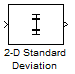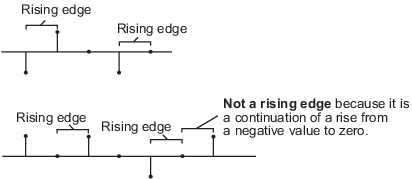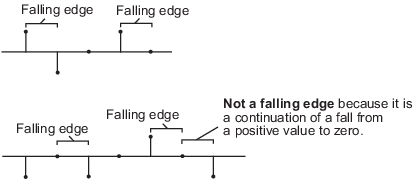# 2-D Standard Deviation

Compute standard deviation of input or sequence of inputs

•Libraries:
Computer Vision Toolbox / Statistics

## Description

The 2-D Standard Deviation block computes the standard deviation of an input array. The input can be a 1-D vector, 2-D matrix, or an N-D-array. The block can compute standard deviation along a specified dimension of the input or the entire input. If you select the Running standard deviation parameter, the block can also track the standard deviation in a sequence of inputs over a time period.

## Ports

### Input

expand all

Input array, specified as a vector, matrix, or N-D- array. This port is unnamed until you select the Enable ROI processing parameter.

Data Types: `single` | `double`

Reset port, specified as a scalar. This port specifies the event that causes the block to reset the running standard deviation. The sample time of the Rst input must be a positive integer and a multiple of the input sample time.

#### Dependencies

To enable this port, select the Running standard deviation parameter and set the Reset port parameter to `Rising edge`, `Falling edge`, `Either edge`, or ```Non-zero sample```.

Data Types: `single` | `double` | `int8` | `int16` | `int32` | `uint8` | `uint16` | `uint32` | `Boolean`

Region of interest (ROI), specified as a four-element vector, m-by-4 matrix, or M-by-N matrix. The input value to this port depends on the ROI type parameter.

Note

You can use the `ROI` port only if the input is a 2-D image.

#### Dependencies

To enable this port, set the Find the standard deviation value over parameter to `Entire input` and select the Enable ROI processing parameter.

Data Types: `single` | `double` | `int8` | `int16` | `int32` | `uint8` | `uint16` | `uint32` | `Boolean` | `fixed point`

Label matrix, specified as a matrix of nonnegative integers. The label matrix represents the objects in an image. The pixels labeled 0 are the background. The pixels labeled 1 make up one object; the pixels labeled 2 make up a second object; and so on. The size of the label matrix must be the same size as the 2-D input.

#### Dependencies

To enable this port, select the Enable ROI processing parameter and set the ROI type parameter to `Label matrix`.

Data Types: `uint8` | `uint16` | `uint32`

Label values of ROIs, specified as a M-element vector. The value of M can be less than or equal to the number of objects that are labeled in the label matrix.

#### Dependencies

To enable this port, select the Enable ROI processing parameter and set the ROI type parameter to `Label matrix`.

Data Types: `uint8` | `uint16` | `uint32`

### Output

expand all

Computed output standard deviation, returned as a scalar, vector, matrix, or N-D-array. The size of the returned output standard deviation depends on the size of the input, and the settings for the Running standard deviation and Find the standard deviation value over parameters.

This port is unnamed until you select the Output flag indicating if ROI is within image bounds and the ROI type parameter is set to `Rectangles`, `Lines`, or `Label matrix`.

#### If Running standard deviation Is Cleared

You can compute standard deviation along any specified dimension of the input or the entire input. The output is a:

• Scalar if the input is of any size and the Find the standard deviation value over parameter is set to `Entire input`.

• Vector if the input is a matrix and the Find the standard deviation value over parameter is set to any one of `Each row`, `Each column`, and `Specified dimension`. In this case, the Dimension value for ```Specified dimension``` can be either 1 or 2.

• Matrix if the input is a 3-D array and the Find the standard deviation value over parameter is set to `Specified dimension` and the Dimension value is 3.

• N-D array if the input is an N-D array and the Find the standard deviation value over parameter is set to an option other than ```Entire input```.

If you compute standard deviation along the Nth dimension of the input, then the returned output is an (N-1)-D array. In this case, the Dimension value for `Specified dimension` is set to N.

Example: For a 3-D input array of size M-by-N-by-P, the dimension of the returned output is:

• 1-by-N-by-P if you set the Find the standard deviation value over parameter to `Entire row`.

• M-by-1-by-P if you set the Find the standard deviation value over parameter to `Entire column`.

• M-by-N if you set the Find the standard deviation value over parameter to `Specified dimension` and the Dimension value to 3.

#### If Running standard deviation Is Selected

When you select the Running standard deviation parameter, the block computes the standard deviation of each sample in the input with respect to all previous samples. The output is of the same size as the input.

Data Types: `single` | `double` | `int8` | `int16` | `int32` | `uint8` | `uint16` | `uint32` | `fixed point`

Validation for ROI, returned as 0 or 1. The output value signifies if all of the ROIs specified at the input lie within the image bounds. The output value depends on the values of the Output and ROI Type parameters.

 ROI Type Output Output from Flag port Description `Rectangles``Lines` ```Individual statistics for each ROI``` 0 ROI is completely outside the input image. 1 ROI is either completely or partially inside the input image. ```Single statistic for all ROIs``` 0 ROI is completely outside the input image. 1 ROI is either completely or partially inside the input image. `Label matrix` ```Individual statistics for each ROI``` 0 Label number is not in the label matrix. 1 Label number is in the label matrix. ```Single statistic for all ROIs``` 0 None of the label numbers are in the label matrix. 1 At least one of the label numbers is in the label matrix.

Note

If the ROI is partially outside the image, the block computes the standard deviation values only for the portion of the ROI that lies within the image bounds.

#### Dependencies

To enable this port, select the Output flag indicating if ROI is within image bounds parameter and set the value of ROI type parameter to `Rectangle`, `Lines`, or ```Label Matrix```.

## Parameters

expand all

Main Tab

Select to track the standard deviation of successive inputs to the block. In this mode, the block treats each element as a channel.

Specify when the block detects a reset event. The block resets the running standard deviation when a reset event is detected at the optional Rst port. The reset sample time must be a positive integer and a multiple of the input sample time.

Specify the reset event as:

• `None` to disable the Rst port.

• `Rising edge` to trigger a reset event when the Rst input does one of the following:

• Rises from a negative value to either a positive value or zero

• Rises from zero to a positive value, where the rise is not a continuation of a rise from a negative value to zero• `Falling edge` to trigger a reset event when the Rst input does one of the following:

• Falls from a positive value to either a negative value or zero

• Falls from zero to a negative value, where the fall is not a continuation of a fall from a positive value to zero• `Either edge` to trigger a reset event when the Rst input is a ```Rising edge``` or `Falling edge`.

• `Non-zero sample` to trigger a reset event at each sample time, when the Rst input is not zero.

Note

When running simulations in the Simulink® multitasking mode, reset signals have a one-sample latency. Therefore, when the block detects a reset event, there is a one-sample delay at the reset port rate before the block applies the reset.

#### Dependencies

To enable this parameter, select the Running standard deviation parameter.

Specify the dimension of the input along which the block computes the standard deviation.

• `Entire input` — Computes standard deviation over the entire input.

• `Each row` — Computes standard deviation over each row.

• `Each column` — Computes standard deviation over each column.

• `Specified dimension`— Computes standard deviation over the dimension specified in the Dimension parameter. By default, the Dimension parameter is set to 1 and the output is the same as when you select ```Each column```.

#### Dependencies

To enable this parameter, clear the Running standard deviation parameter.

Specify the dimension of the input array over which the standard deviation is computed as a one-based value. The value of this parameter is set to 1..

#### Dependencies

To enable this parameter, set the Find the standard deviation value over parameter to `Specified dimension`.

ROI Processing

Select to calculate standard deviation within a particular ROI in the image.

Note

Full ROI processing is available only if you have a Computer Vision Toolbox™ license. If you do not have a Computer Vision Toolbox license, you can still use ROI processing, but the ROI type is limited to `Rectangles`.

#### Dependencies

To enable this parameter, set the Find the standard deviation value over parameter to `Entire input`.

Specify the type of ROI that represents the regions in the image over which the block computes the standard deviation. The type of ROI can be a rectangle, line, label matrix, or a binary mask.

 Parameters Description ROI type Inputs to the ROI port `Rectangles` Four-element row vector ```[x y width height]```m-by-4 matrix `$\left[\begin{array}{cccc}{x}_{1}& {y}_{1}& widt{h}_{1}& heigh{t}_{1}\\ {x}_{2}& {y}_{2}& widt{h}_{2}& heigh{t}_{2}\\ ⋮& ⋮& ⋮& ⋮\\ {x}_{M}& {y}_{M}& widt{h}_{M}& heigh{t}_{M}\end{array}\right]$`,where m is the number of rectangles. Each row of the matrix corresponds to a different rectangle. `x` and `y` are the one-based coordinates of the upper left corner of the rectangle.`width` and `height` are the width and height, in pixels, of the rectangle. The values of `width` and `height` must be greater than 0. `Lines` Four-element row vector ```[x1 y1 x2 y2]```m-by-4 matrix `$\left[\begin{array}{cccc}{x}_{11}& {y}_{11}& {x}_{12}& {y}_{12}\\ {x}_{21}& {y}_{21}& {x}_{22}& {y}_{22}\\ ⋮& ⋮& ⋮& ⋮\\ {x}_{M1}& {y}_{M1}& {x}_{M2}& {y}_{M2}\end{array}\right]$`,where m is the number of lines. Each row of the matrix corresponds to a different line. `x1` and `y1` are the coordinates of the beginning of the line.`x2` and `y2` are the coordinates of the end of the line. `Label matrix` M-by-N matrix Matrix of the same size as the input image. The matrix contains label values that represent different objects in an image. The pixels labeled 0 are the background. The pixels labeled 1 make up one object; the pixels labeled 2 make up a second object; and so on. `Binary mask` M-by-N matrix Matrix of the same size as the input image. The binary mask classifies image pixels as belonging to either the region of interest or the background. The mask pixel values of 1 indicate that the image pixel belongs to the ROI. The mask pixel values of 0 indicate that the image pixel is part of the background.

#### Dependencies

To enable this parameter, select the Enable ROI processing parameter.

Specify the portion of the ROI from which the block has to calculate 2-D standard deviation. The ROI portion is either the entire ROI or the ROI perimeter.

#### Dependencies

To enable this parameter, select the Enable ROI processing parameter and set the ROI type parameter to `Rectangles`.

Specify whether to calculate 2-D standard deviation individually for each ROI or for the entire ROI.

• If you select `Individual statistics for each ROI`, the block outputs a vector of standard deviation values. The size of the output vector is equal to the number of ROIs.

• If you select `Single statistic for all ROIs`, the block outputs a scalar value. The scalar value represents the statistical value for all the specified ROIs.

#### Dependencies

To enable this parameter, select the Enable ROI processing parameter and set the ROI type parameter to `Rectangles`, `Lines`, or `Label matrix`.

Select to expose the Flag port. For a description of the Flag port output, see Flag.

#### Dependencies

To enable this parameter, select the Enable ROI processing parameter and set the ROI type parameter to `Rectangles`, `Lines`, or `Label matrix`.

Data Types Tab

For details on the fixed-point block parameters, see Specify Fixed-Point Attributes for Blocks (DSP System Toolbox).

Select this parameter to prevent the fixed-point tools from overriding the data types you specify in this block. For more information, see Lock the Output Data Type Setting (Fixed-Point Designer).

## Block Characteristics

 Data Types `double` | `single` Multidimensional Signals `no` Variable-Size Signals `yes`

## Version History

Introduced in R2011b